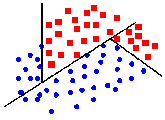# Pattern Separation via Mathematical ProgrammingThis WWW page describes work in Pattern Separation via Linear Programming in the Mathematical Programming section of the University of Wisconsin-Madison Computer Sciences Department.

# Brief History and Method Outline

Mathematical optimization approaches, in particular linear programming, have long been used in problems of pattern separation. In  linear programs were used to construct planes to separate linearly separable point sets. Separation by a nonlinear surface using linear programming was also described, whenever the surface parameters appeared linearly, e.g. a quadratic or polynomial surface. These formulations however could fail on sets that were not separable by a surface linear in its parameters.

A Multisurface Method (MSM) [68,93] avoided this difficulty. MSM separates 2 disjoint finite point sets in n-dimensional Euclidean space as follows:

1. Choose 2 parallel planes in n-dimensional Euclidean space as close together so that only the region between the two planes contains points from both sets (i.e. the regions NOT between the 2 parallel planes contain only points of 1 set or no points).
2. Discard the points in the regions not between the 2 parallel planes.
3. Repeat the process on the points between the 2 parallel planes, until the region between the 2 parallel planes contains no points or very few points.

Multisurface Method Tree (MSM-T), a variant on the Multisurface Method was developed in [92a], [92b], . Let A and B be finite disjoint point sets in n-dimensional Euclidean space. The goal of MSM-T is to determine a sequence of planes in n-dimensional Euclidean space that separate the sets A and B as follows:

1. Determine a plane in n-dimensional Euclidean space that minimizes the average "distances" of misclassified points. A point from set A is misclassified if it lies on the side of the separating plane assigned to B. Similarly, a point from set B is misclassified if it lies on the side of the separating plane assigned to A.
2. If the regions assigned to A and B contain only (or mostly) points of the set A or B, then stop. Otherwise, generate another error-minimizing plane (in 1.) in this region.
The sequence of planes generated can be viewed as a decision tree. For each node in the tree, the best split of the points reaching that node is found by solving the LP in 1, above. The node is split into 2 branches, and the same procedure is applied until there are only (or mostly) points of one set at the node. This linear programming approach can also be viewed as training a neural network with 1 hidden layer (see ).

MSM-T has been shown to learn concepts as well or better than more traditional learning methods such as C4.5 and CART. It also has an advantage over artificial neural network (ANN) methods such as backpropagation in that training proceeds much faster (see [92a]).

# Implementations of MSM-T

MSM-T has been implemented in C using the MINOS numerical optimization package by Nick Street and Kristin Bennett. MSM-T has also been implemented for the MATLAB optimization package by Paul Bradley. Following is a description of the MATLAB implementation of MSM-T. Together with the M-files required to run it.

## Chronological Bibliography

 O. L. Mangasarian.
Linear and Nonlinear Separation of Patterns by Linear Programming. Operations Research, Vol. 13, No. 3, May-June, 1965, pages 444 - 452.
 O. L. Mangasarian.
Multisurface Method of Pattern Separation. IEEE Transactions on Information Theory, Vol. IT-14, No. 6, November 1968, pages 801 - 807.
[92a] K. P. Bennett.
Decision Tree Construction via Linear Programming. Proceedings of the 4th Midwest Artificial Intelligence and Cognitive Science Society Conference, 1992, pages 97 - 101.
[92b] K. P. Bennett and O. L. Mangasarian.
Robust Linear Programming Discrimination of Two Linearly Inseparable Sets. Optimization Methods and Software, Vol. 1, 1992, pages 23 - 34.
 O. L. Mangasarian.
Mathematical Programming in Neural Networks. ORSA Journal on Computing, Vol. 5, No. 4, Fall 1993, pages 349 - 360.

Last modified: Wed Jul 12 10:40:37 1995 by Paul Bradley
paulb@cs.wisc.edu